MCAT Physical : Optics

Example Questions

Example Question #1 : Combining Lenses

A compound microscope consists of an eyepiece with an angular magnification of 25 and an objective lens of unknown focal length. If the length of the microscope tube is 25cm, what magnitude of objective focal length is necessary to achieve an overall magnification of 500?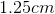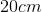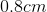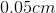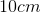Explanation:

Relevant equations: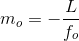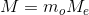= length of microscope tube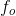= focal length of objective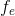= focal length of eyepiece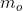= linear magnification of objective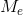= angular magnification of eyepiece= total magnification of microscope

Given: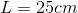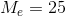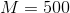Step 1: Plug the expression forinto the equation for total magnification,.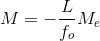Step 2: Rearrange to isolate the unknown,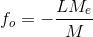Step 3: Plug in given quantities, taking the absolute value to find the magnitude of.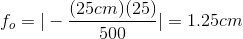Example Question #12 : Mirrors And Lenses

What diameter of telescope objective lens is necessary to resolve two stars that primarily emit 600nm light and have an angular separation of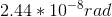?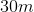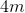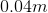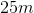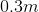Explanation:

Relevant equations: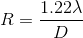= angular separation of sources, in radians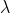= wavelength of light emitted by sources, in meters= diameter of telescope

Step 1: Rearrange equation to isolate the unknown,: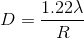Step 2: Plug in the given numbers for wavelength and angular separation: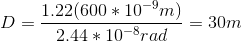Example Question #1 : Types Of Image

A man stands ten meters away from a converging mirror with a focal length of two meters. What is true of the image he sees?

His image is virtual, upright, and magnified

His image is virtual, upright, and minimized

His image is real, upright, and magnified

His image is real, inverted, and magnified

His image is real, inverted, and minimized

His image is real, inverted, and minimized

Explanation:

The first thing to consider when answering this question is the fact that real images are always inverted and virtual images are always upright. Once you have determined one or the other, two answer choices can be eliminated.

The first equation that is necessary for this question is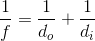.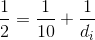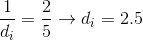From this we can determine that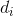is equal to. Sinceis a positive number we know the image is real, and thus inverted.

The second equation to consider is for magnification:.

If the absolute value ofis greater than one, the image is magnified, and if the value is less than one, it is minimized.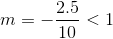We would expect the image to be minimized.

Example Question #1 : Types Of Image

For a nearsighted person, the image of a distant object is formed __________.

behind the retina, and corrected using a diverging lens

in front of the retina, and corrected using a diverging lens

in front of the retina, and corrected using a converging lens

behind the retina, and corrected using a converging lens

in front of the retina, and corrected using a diverging lens

Explanation:

In nearsightedness, the person cannot see far objects due to increased refraction. This causes the image to be formed in front of the retina. This condition is corrected using a diverging lens to compensate for the "over refraction" by the deformed cornea.

Example Question #1 : Types Of Image

An object is placed 50cm in front of a converging lens whose focal length is 20cm. Which of the following best describes the image that is formed?

Larger than the object and real

Smaller than the object and real

The same size as the object and virtual

Smaller than the object and virtual

Larger than the object and virtual

Smaller than the object and real

Explanation:

Relevant equations: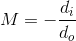Step 1: Plug in the given focal length and object distance to find the image distance: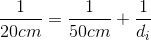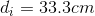Step 2: Find the magnification and orientation of the image: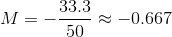A negative magnification means the image is inverted, and therefore real. A magnification smaller than 1 means the image is smaller than the object.

The following result is true, in general, for converging lenses: if the object is outside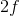, then the image is inverted, real, and smaller than the object.

Example Question #11 : Mirrors And Lenses

Jimmy is farsighted and uses a convex lens to correct his vision. Wendy is nearsighted and uses a concave lens to correct her vision. They both wear glasses. During a camping trip, they notice they do not have any matches, and decide to use their glasses to start the fire. Whose glasses could be used to start the fire?

Wendy

Neither glasses would work

Jimmy

Both glasses would work equally well

Jimmy

Explanation:

This question deals with an application of optics. In this case we have a farsighted person and a near sighted person. The farsighted person would use a convex lens, which is a converging lens. This would allow all of the rays of light to converge on a single point, allowing them to heat the object up and start a fire. Wendy’s glasses are diverging lenses, which would cause the rays to separate.

Example Question #1 : Reflection And Refraction

A light ray traveling through a medium is reflected by a second medium at an angle of 20⁰ to the interface between the two media.  Which of the following is true?

The frequency of the light wave is greater upon reflection

The refractive index of the second medium is equal to sin(20⁰)

The angle of reflection is 70o

The refractive indices of the media must be equal

The angle of reflection is 70o

Explanation:

The angle of reflection is the angle between the reflected light ray and a line perpendicular to the interface between the two media. The angle of reflection must be complementary to 20o.

90o – 20o = 70⁰

Example Question #2 : Reflection And Refraction

An incandescent light bulb is shown through a glass prism. The certain wavlength of the light is then directed into a glass cuvette containing an unknown concentration of protein. Commonly, this process is called spectroscopy and is used to determine the concentrations of DNA, RNA, and proteins in solutions. The indices of reflection of air, glass, and the solution are 1, 1.5, and 1.3, respectively.

The process of light entering glass from the air is called __________.

absorption

refraction

diffraction

reflection

refraction

Explanation:

This question is asking us about the different processes that can happen to light upon hitting a surface. Thinking back to the properties of light, we know that absorption, reflection, and refraction are all processes that light can undergo when interacting with a surface.

Absorption means that the energy associated with the light is captured, and no photons are ejected from the surface after the collision of the incident photon and the surface. Reflection occurs when no light enters the new medium and instead bounces off at the angle to normal that it hit. Refraction occurs when some light enters the new medium. In this case, light is entering glass from the air; thus, the process we are concerned about is refraction.

Example Question #3 : Reflection And Refraction

Sound projected from an opera stage strikes a flat wall in the opera house at an angle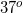to the normal. What conclusion can be drawn about the reflection of this sound from the wall back into the room?

The reflection will be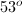to the opposite side of the normal

The reflection will be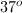to the same side of the normal

The reflection will beto the same side of the normal

The reflection will be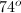to the opposite side of the normal

The reflection will beto the opposite side of the normal

The reflection will beto the opposite side of the normal

Explanation:

For all waves, the angle of incidence is equal to the angle of reflection. The projection is to the opposite side of the normal at the same angle as the incident wave.

Think of the wall as a mirror. The angle with which the wave impacts a mirror will be equal to the angle with which it is reflected, but mirrored across the normal.

Example Question #1 : Reflection And Refraction

Glass has an index of refraction of roughly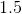. How much time would it take for light to pass through this glass if it were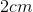thick?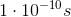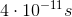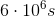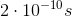Explanation:

Since the index of refraction is 1.5, we can determine the speed of light in the glass using the following equation: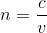Rearranged to solve for velocity: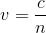Once the speed of light in the glass is known, we can use this quantity to determine how long it will take for the light to travel the width of the glass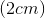.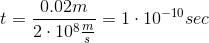All MCAT Physical Resources